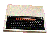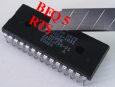×   Main Menu ALL The 8BS News Manuals (New menu) Links Worth a Look Tools Disc and Basic Webring Site Map 8BS Guestbook Old Guest Book Me The Barnsley Rovers# The BBC and Master Computer Public Domain Library

### A8A1 ASN

Submitted by Steve Fewell

Description:

Call routine &96DA to get the numeric value (at the current position of the BASIC text pointer) and convert it to a
Floating-Point value (if it was an Integer).

If the FWA sign is positive then jump to &A8AF to calculate the ASN result and exit.

If the FWA sign is negative then:
* Clear the FWA Sign byte (to make the FWA value positive)
* Call routine &A8AF to calculate the ASN result for the FWA value
* Jump to &A8D2 to store A (#&FF) in the FWA sign byte (to make the FWA value negative) and exit

[A8AF] Calculate ASN result:
Call routine &A50D to store the FWA value in temporary Floating-point location &0476-&047A.
Call routine &A929 to:
* FWA = FWA * FWA (calculate the square of FWA)
* FWA = 1.0 [&BF92] - FWA
* FWA = SQR(FWA) (calculate the square root of FWA)
If the FWA Mantissa byte 1 is zero then jump to &A896 to exit with the FWA set to the floating-point constant at
location &BF2E [which is 1.57079633].

Call &A5B3 to set the FWA = (as the result is a Floating-Point value).
Jump to &A8C6 to set the FWA to the Arc-Tangent of the FWA value [FWA = ATN(FWA)].

The ASN calculation for a non-zero positive value is therefore:
ASN(x) = ATN(x / SQR(1 - (x * x)))

Example 1: ASN(0)
[A8AF] Store FWA in &0476
Store FWA in &046C
FWA = FWA * &046C = 0.0
FWA = 1.0 - FWA = 1.0
FWA = SQR(FWA) = 1.0
FWA = &0476 (0) / FWA = 0.0
FWA = ATN(FWA) = 0.0

Example 2: ASN(0.25)
[A8AF] Store FWA in &0476
Store FWA in &046C
FWA = FWA * &046C = 0.0625
FWA = 1.0 - FWA = 0.9375
FWA = SQR(FWA) = 0.9682458
FWA = &0476 (0.25) / FWA = 0.2581988
FWA = ATN(FWA) = 0.2526802

Example 3: ASN(-0.75)
[A8A4] Sign byte of FWA is negative, so clear the sign of the FWA = 0.75
[A8AF] Store FWA in &0476
Store FWA in &046C
FWA = FWA * &046C = 0.5625
FWA = 1.0 - FWA = 0.4375
FWA = SQR(FWA) = 0.6614378
FWA = &0476 (0.75) / FWA = 1.1338934
FWA = ATN(FWA) = 0.848062
[A8AD] Store A (#&FF) in the FWA sign byte. FWA = -0.848062

This diagram shows the relationship between the trig functions.

### Disassembly for the ASN routine

 A8A1 032 218 150 20 DA 96 JSR &96DA Get and Check Float (convert if Int) A8A4 . 165 046 A5 2E LDA &2E A8A6 016 007 10 07 BPL 7 --> &A8AF A8A8 d. 100 046 64 2E STZ &2E A8AA 032 175 168 20 AF A8 JSR &A8AF A8AD # 128 035 80 23 BRA 35 --> &A8D2 Store A in location &2E (i.e. make result negative) and exit A8AF 032 013 165 20 0D A5 JSR &A50D Store FWA to &0476 and set argp to &0476 A8B2 ) 032 041 169 20 29 A9 JSR &A929 A8B5 1 165 049 A5 31 LDA &31 A8B7 240 005 F0 05 BEQ 5 --> &A8BE A8B9 032 179 165 20 B3 A5 JSR &A5B3 FWA=&0476/FWA & exit with A=#&FF A8BC 128 008 80 08 BRA 8 --> &A8C6 Calculate ATN result [FWA=ATN(FWA)] A8BE . 169 046 A9 2E LDA#&2E A8C0 L 076 150 168 4C 96 A8 JMP &A896 Load FWA with FP constant at &BF00 + AOr